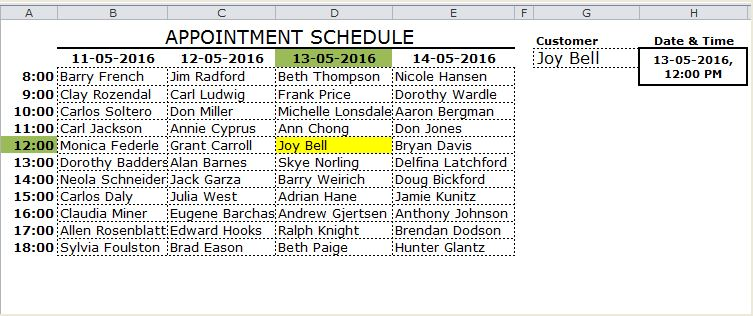#### Email Us

Follows

In my research and tutoring excel, I have found that many people find the Reverse lookup concept to be among the top 10 complicated things in excel. This article hopes to shed more light than heat in demystifying the Reverse Lookup in excel.

In the normal lookup, we use the Column and/or Row header to return the value that falls in the intersection. But in the reverse lookup, we shall use the value to return the column and/or row header

For example, say you have below appointments Calendar and you want to lookup &  return the scheduled date & time for customer Joy BellThere are 4 ways to carry out this kind of a Reverse Lookup;

## USING INDEX & SUMPRODUCT

```=INDEX(D3:G3,0,
SUMPRODUCT(--(D4:G14=I3)*(COLUMN(D3:G3)-COLUMN(D3)+1)))
+INDEX(C4:C14,
SUMPRODUCT(--(D4:G14=I3)*(ROW(C4:C14)-ROW(C4)+1)))```

### How it works:

The trick is to break down the formula into small parts;

1. INDEX(D3:G3,0,SUMPRODUCT(–(D4:G14=I3)*(COLUMN(D3:G3)-COLUMN(D3)+1))) this is the part that looks up the Date values in the columns

►SUMPRODUCT(–(D4:G14=I3)*(COLUMN(D3:G3)-COLUMN(D3)+1))  returns a column number given a criteria i.e.

• – -(D4: G14=I3) →returns an array of 1 & 0. 1 represents where the criterion is met
```{0,0,0,0;0,0,0,0;0,0,0,0;0,0,0,0;0,0,1,0;
0,0,0,0;0,0,0,0;0,0,0,0;0,0,0,0;0,0,0,0;0,0,0,0}```
• (COLUMN(D3: G3)-COLUMN(D3)+1)→ returns an array of relative positions of the columns
` {1,2,3,4}`

SUMPRODUCT takes up the 2 arrays and  using the techniques of conjunction truth table returns 3

►INDEX(D3:E3,0,3) returns the value in column 3 given the range D3:E3= 13-05-2016

2. INDEX(C4:C14,SUMPRODUCT(–(D4:G14=I3)*(ROW(C4:C14)-ROW(C4)+1)) this is the part that looks up the Time values in the rows.

The breakdown of the formula is the same as explained above other than INDEX looks up the rows instead of columns.

When you combine the two parts you form a DateTime Value which you can custom format to fit.

`=INDEX(D3:E3,0,3)+INDEX(C4:C14,5) = 13-05-2016  12:00:00 PM`

## 2.Using an Array Formula (INDEX & MAX)

```{=INDEX(D3:G3,
MAX((D4:G14=I7)*(COLUMN(D3:G3)-COLUMN(D3)+1)))
+INDEX(C4:C14,
MAX((D4:G14=I7)*(ROW(C4:C14)-ROW(C4)+1)))}```

### How it works:

1. =INDEX(D3: G3,MAX((D4: G14=I7)*(COLUMN(D3: G3)-COLUMN(D3)+1))) like in the SUMPRODUCT formula, this is the part that looks up the Date Value

(D4: G14=I7)*(COLUMN(D3: G3)-COLUMN(D3)+1) this part generates an array of Column number where the criteria are met and zero for the rest.

```{0,0,0,0;0,0,0,0;0,0,0,0;0,0,0,0;0,0,3,0
;0,0,0,0;0,0,0,0;0,0,0,0;0,0,0,0;0,0,0,0;0,0,0,0}```

MAX function returns the  maximum number in the array

```MAX({0,0,0,0;0,0,0,0;0,0,0,0;0,0,0,0;0,0,3,0
;0,0,0,0;0,0,0,0;0,0,0,0;0,0,0,0;0,0,0,0;0,0,0,0})=3```

INDEX(D3:E3,0,3) returns the Date Value.

1. INDEX(C4:C14,MAX((D4:G14=I7)*(ROW(C4:C14)-ROW(C4)+1))) this generates the Time Value

The same breakdown works with the Time Value.

NB: This is an Array formula so remember to Ctrl + Shift +Enter

## 3. Using Array Formula (SMALL & IF)

```{=SMALL(
IF(D4:G14=I10,(D3:G3+C4:C14))
,1)}```

This is the shortest and easiest to understand.

First, you need to understand how Excel stores date and time i.e. dates as serial numbers and time as a fractional portion of a 24 hour day.

Secondly, you need to understand that a  DateTime Value is just a sum of this Date serial Number and fractional Time Value.

►(D3:G3+C4: C14) returns a two-dimensional array of DateTime Value

```{42501.3333333333,42502.3333333333,42503.3333333333,42504.3333333333;
42501.375,42502.375,42503.375,42504.375;42501.4166666667,42502.4166666667,
42503.4166666667,42504.4166666667;42501.4583333333,42502.4583333333,
42503.4583333333,42504.4583333333;42501.5,42502.5,42503.5,42504.5;
42501.5416666667,42502.5416666667,42503.5416666667,42504.5416666667;
42501.5833333333,42502.5833333333,42503.5833333333,42504.5833333333;
42501.625,42502.625,42503.625,42504.625;42501.6666666667,42502.6666666667,
42503.6666666667,42504.6666666667;42501.7083333333,42502.7083333333,
42503.7083333333,42504.7083333333;42501.75,42502.75,42503.75,42504.75}```

►IF(D4: G14=I10,(D3:G3+C4: C14)) returns an array of the DateTime value that meets the criteria

```{FALSE,FALSE,FALSE,FALSE;FALSE,FALSE,FALSE,FALSE;FALSE,FALSE,FALSE,
FALSE;FALSE,FALSE,FALSE,FALSE;FALSE,FALSE,42503.5,FALSE;FALSE,FALSE,
FALSE,FALSE;FALSE,FALSE,FALSE,FALSE;FALSE,FALSE,FALSE,FALSE;FALSE,FALSE,
FALSE,FALSE;FALSE,FALSE,FALSE,FALSE;FALSE,FALSE,FALSE,FALSE}```

►SMALL returns the first smallest number in the array =42503.5

►Format the number “dd-mm-yyyy h:mm AM/PM” = 13-05-2016 12:00PM

## 4. Using  AGGREGATE

Other than INDEX & SUMPRODUCT combination this is the other NON-Array formula you can use to do this Reverse lookup

`=AGGREGATE(15,6,((D3:G3+C4:C14)/(D4:G14=I14)),1)`

### How it works;

Watch these Videos on the AGGREGATE Function.